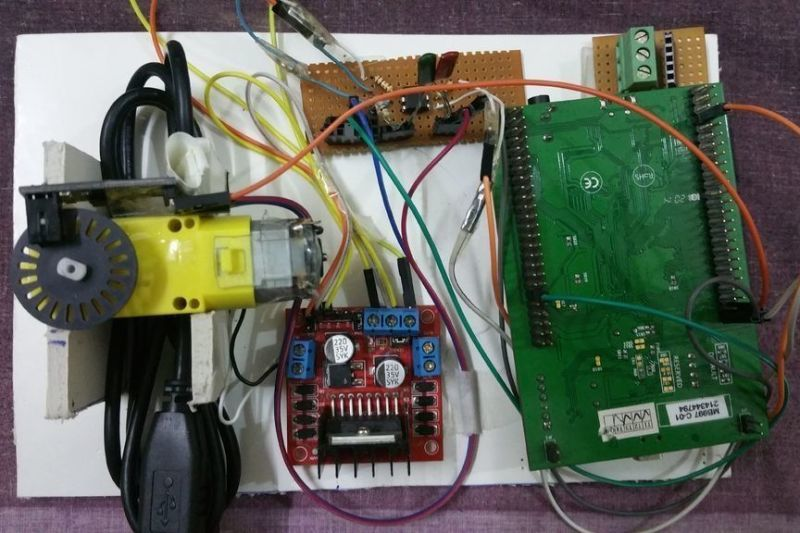Using PID Controller.

Following are the two main methods to control the speed of dc motor

a) Controlling the voltage manually:

In industrial applications, it is essential to have some mechanism of speed control of DC Motor. At times, we may require a higher speed than normal or a lower speed than normal. Therefore, we need good and eﬃcient methods of speed control. One of the crudest methods of speed control is by controlling the supply voltage. We can vary the voltage to vary the speed.

b) Controlling the PWM using PID:

Another more eﬃcient method is by using a microcontroller. The DC Motor is connected to the microcontroller via a Motor Driver. The Motor Driver is an IC which receives a PWM( Pulse Width Modulation) input from the microcontroller and gives an output to the DC Motor based on the input. Figure 1.2: PWM Signal Chapter 1. Introduction 3 The working of PWM can be explained by ﬁrst considering a PWM signal. It consists of continuous pulses of a certain time period. The time period is the time taken by a point to travel the distance equal to one wavelength. These pulses can only have binary values (HIGH or LOW). We also have two other quantities, namely pulse width and duty cycle. Pulse width is the time for which the output of the PWM is HIGH. The duty cycle is the percentage of the pulse width to the time period. For the rest of the time of the time period, the output is LOW. The duty cycle controls the speed of the motor directly. If in one time period, the DC Motor is provided a positive voltage for a certain time, it will move at a certain speed. If the positive voltage is provided for some more time, the speed will be larger. So in eﬀect, by varying the pulse width, the duty cycle of the PWM can be varied. By varying the duty cycle of the DC Motor, the speed of the motor can be varied. Speed Control of a DC Motor

PROBLEM:

The problem with the ﬁrst method of speed control was that the voltage can have variations with respect to time. These variations mean the speed is not uniform. Therefore, the ﬁrst method is undesirable.

SOLUTION:

We use the second method to control the speed. We supplement the second method using a PID Algorithm.

I BUILT MY SITE FOR FREE USING